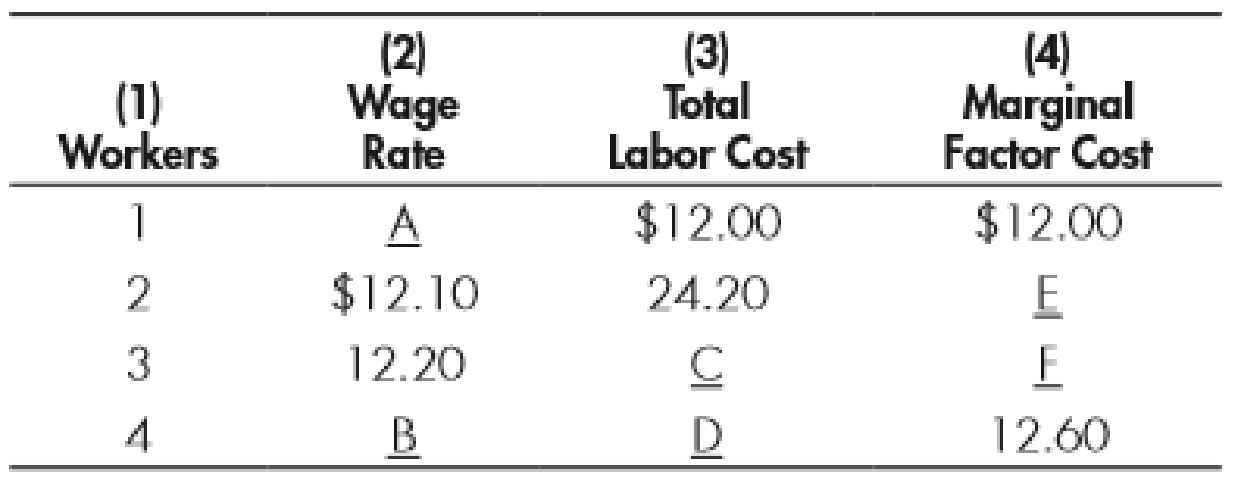Chapter 27, Problem 1WNG

Chapter
Section
Textbook Problem

Determine the appropriate numbers for the lettered spaces:To determine

Identify the values of wage rates, total labor cost (TLC), and marginal factor cost (MFC).

Explanation

Total labor cost can be calculated using the formula given below:

Total labor cost=Wage rate ×Workers        (1)

Marginal factor cost can be calculated using the formula given below:

Marginal factor cost=Changes in total labor costChanges in workers        (2)

Since the wage rate paid for 3 workers is $12.20 and the wage rate paid for 2 workers is$12.10, the difference between wage rates is $0.10 (12.20-12.10), when an additional labor is hired. Thus, the wage rate at point A can be calculated as follows: Wage rateA=Wage rateBWage rate difference=$12.10$0.10=$12.10$0.10=$12

Thus, at point A, the wage rate is $12. The wage rate at point B can be calculated as follows: Wage rateA=Wage ratec+Wage rate difference=$12

Still sussing out bartleby?

Check out a sample textbook solution.

See a sample solution

The Solution to Your Study Problems

Bartleby provides explanations to thousands of textbook problems written by our experts, many with advanced degrees!

Get Started

Find more solutions based on key concepts